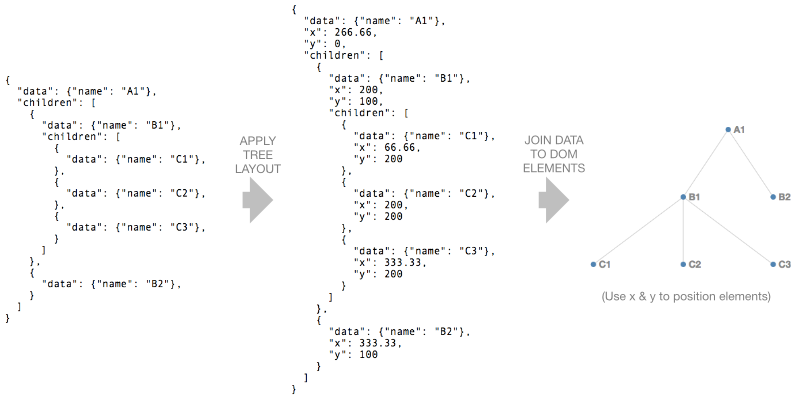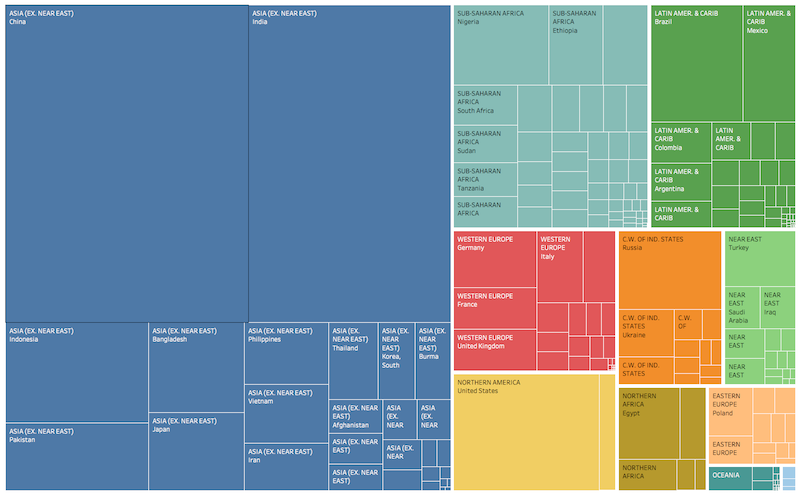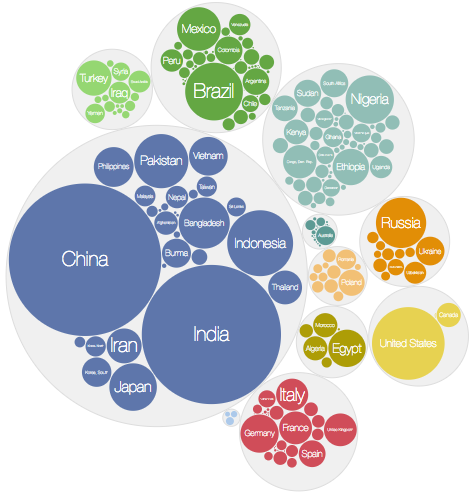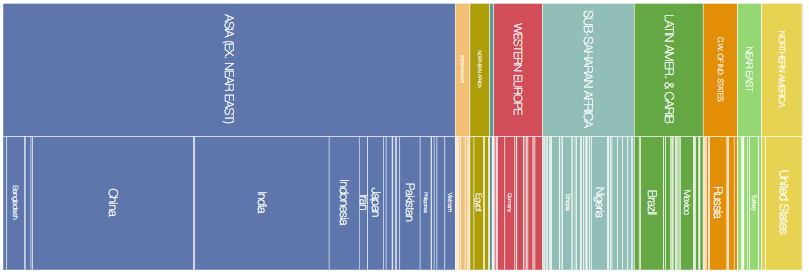D3 in Depth covers version 6 of D3
Build a real-world, custom, interactive and beautiful data visualization from scratch using D3.
Over 50 online text-based lessons on D3, interaction, code architecture, state management, styling and more.
Visit course page

Layouts

packed circles:

and network graphs:

In essence a layout is just a JavaScript function that takes your data as input and adds visual variables such as position and size to it.

For example the tree layout takes a hierarchical data structure and adds x and y values to each node such that the nodes form a tree-like shape:D3 has a number of hierarchy layouts for dealing with hierarchical (or tree) data as well as a chord layout (for network flows) and a general purpose force layout (physics-based simulation). We’ll cover the force layout in a separate chapter.

(It’s also possible to create your own layouts. For example a simple function that adds positional information to an array of data can be considered a layout.)

Hierarchical layouts

D3 has a number of hierarchical layouts to help with visualising hierarchies (or trees) e.g.

In this section we’ll look at the tree, cluster, treemap, pack and partition layouts. Note that treemap, pack and partition are designed to lay out hierarchies where the nodes have an associated numeric value (e.g. revenue, population etc.).

D3 version 4 requires the hierarchical data to be in the form of a d3.hierarchy object which we’ll cover next.

d3.hierarchy

A d3.hierarchy object is a data structure that represents a hierarchy. It has a number of functions defined on it for retrieving things like ancestor, descendant and leaf nodes and for computing the path between nodes. It can be created from a nested JavaScript object such as:

Typically you don’t need to operate on the hierarchy object itself but there are some useful functions defined on it such as:

root.descendants() returns a flat array of root’s descendants and root.links() returns a flat array of objects containing all the parent-child links.

More examples of hierarchy functions

We’ll now look at the tree, cluster, treemap, pack and partition layouts.

tree layout

The tree layout arranges the nodes of a hierarchy in a tree like arrangement.

We start by creating the tree layout using:

We can configure the tree’s size using .size:

We can then call treeLayout, passing in our hierarchy object root:

This’ll write x and y values on each node of root.

We can now:

• use root.descendants() to get an array of all the nodes
• join this array to circles (or any other type of SVG element)
• use x and y to position the circles

and

• join the array to line (or path) elements
• use x and y of the link’s source and target to position the line

(In the case of root.links() each array element is an object containing two properties source and target which represent the link’s source and target nodes.)

cluster layout

The cluster layout is very similar to the tree layout the main difference being all leaf nodes are placed at the same depth.

treemap layout

Treemaps were invented by Ben Shneiderman to visually represent hierarchies where each item has an associated value.

For example, we can think of country population data as a hierarchy where the first level represents the region and the next level represents each country. A treemap will represent each country as a rectangle (sized proportionally to the population) and group each region together:D3’s treemap layout is created using:

As usual we can configure our layout e.g.

Before applying this layout to our hierarchy we must run .sum() on the hierarchy. This traverses the tree and sets .value on each node to the sum of its children:

Note that we pass an accessor function into .sum() to specify which property to sum.

We can now call treemapLayout, passing in our hierarchy object:

The layout adds 4 properties x0, x1, y0 and y1 to each node which specify the dimensions of each rectangle in the treemap.

Now we can join our nodes to rect elements and update the x, y, width and height properties of each rect:

If we’d like labels in each rectangle we could join g elements to the array and add rect and text elements to each g:

treemap layouts can be configured in a number of ways:

• the padding around a node’s children can be set using .paddingOuter
• outer and inner padding can be set at the same time using .padding

Treemaps can use different tiling strategies and D3 has several built in (treemapBinary, treemapDice, treemapSlice, treemapSliceDice, treemapSquarify) and the configuration function .tile is used to select one:

treemapBinary strives for a balance between horizontal and vertical partitions, treemapDice partitions horizontally, treemapSlice partitions vertically, treemapSliceDice alternates between horizontal and vertical partioning and treemapSquarify allows the aspect ratio of the rectangles to be influenced.

The effect of different squarify ratios can be seen here.

pack layout

The pack layout is similar to the tree layout but circles instead of rectangles are used to represent nodes. In the example below each country is represented by a circle (sized according to population) and the countries are grouped by region.D3’s pack layout is created using:

As usual we can configure its size:

As with the treemap we must call .sum() on the hierarchy object root before applying the pack layout:

The pack layout adds x, y and r (for radius) properties to each node.

Now we can add circle elements for each descendant of root:

Labels can be added by creating g elements for each descendant:

partition layout

The partition layout subdivides a rectangular space into a layer for each layer of the hierarchy. Each layer is subdivided for each node in the layer:D3’s partition layout is created using:

As usual we can configure its size:

As with the treemap we must call .sum() on the hierarchy object root and before applying the partition layout:

The partition layout adds x0, x1, y0 and y1 properties to each node.

We can now add rect elements for each descendant of root:

If we’d like to change the orientation of the partition layout so that the layers run left to right we can swap x0 with y0 and x1 with y1 when defining the rect elements:

We can also map the x dimension into a rotation angle and y into a radius to create a sunburst partition:

chord layout

Chord diagrams visualise links (or flows) between a group of nodes, where each flow has a numeric value. For example, they can show migration flows between countries. (Personally I find them difficult to interpret!)

The data needs to be in the form of an n x n matrix (where n is the number of items):

The first row represents flows from the 1st item to the 1st, 2nd and 3rd items etc.

We create the layout using:

and we configure it using .padAngle() (to set the angle between adjacent groups in radians), .sortGroups() (to specify the order of the groups), .sortSubgroups() (to sort within each group) and .sortChords() to determine the z order of the chords.

We apply the layout using:

which returns an array of chords. Each element of the array is an object with source and target properties. Each source and target has startAngle and endAngle properties which will define the shape of each chord.

We use the ribbon shape generator which converts the chord properties into path data (see the Shapes chapter for more information on shape generators).